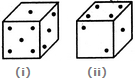# Non Verbal Reasoning - Cubes and Dice - Discussion

### Discussion :: Cubes and Dice - Section 2 (Q.No.33)

33.

Two positions of a dice are shown below. When there are two dots at the bottom, the number of dots at the top will be[A]. 3 [B]. 5 [C]. 6 [D]. Cannot be determined

Explanation:

Number 1 is common to both the positions of the dice. We assume the dice in fig. (ii) to be rotated so that 1 dot moves to the top face (i.e. face V as per activity 1) i.e. to the same position as in fig. (i) and 2 and 4 dots move to the faces hidden behind the faces with 3 and 5 dots respectively. Thus, the combined figure will have 1 dot on the Top (i.e. on face V), 5 dots on Front face (i.e. on face I), 3 dots on RHS face (i.e. face II), 4 dots on the Rear face (i.e. face III) and 2 dots on the LHS face (i.e. face IV). Clearly, 3 dots lie on the face opposite the face having 2 dots. Therefore, when there are 2 dots at the bottom, the number of dots at the top will be 3.

 X Ranjan said: (Feb 14, 2016) Both dice are standard dice how can possible to opposite 3?

 Dharmajeet said: (Feb 3, 2017) Not understood, I think opposite number sum is 7 but is it not.

 Ansab said: (Jul 8, 2017) According to me, it is 5 not 3.

 Damian said: (Aug 31, 2017) In my opinion, it should be 5.

 Raizada said: (May 15, 2018) Yes, it is 5 only.

 Joyita said: (Aug 9, 2019) It is a standard dice so the sum of opposite face of the dice is 7. Answer is 5.